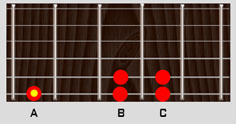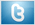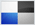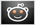# Taking Scale Analyzing A Step Further (part III)

This time around we will cover some tricks and techniques used to make analyzing quicker and easier.

It is very important to learn the different techniques you have at your disposal to determine the proper scale to use. If the intervals for these scales are always W, W, H, W, W, W, H, then no matter where you start or stop (playing other modes), you can see a few things that will always be true.

• 1. One of the techniques you would use is to look for the half steps. Half steps and where they appear can tell you a lot about what mode you are looking at. The reason for this is that there are only two half steps in the traditional modes. When dealing with the traditional modes, there are never two half steps next to each other, as a matter of fact there are always at least two whole steps between them. As you can see in the intervals, either of the two half steps will have either two or three whole steps next to them no matter how you slice it. This means any time we see a half step we can safely add two whole steps before and after it. This can often point out notes that we did not already know belonged to the scale.
• 2. As you already know from learning the intervals, we never use more than a whole step in the traditional modes either. This further agrees with what we just learned above, and if you have a half step it is sure to be preceded and followed by a whole step (two actually).
• 3. Another important technique that can help you analyze more quickly is to look for "spoiler" notes. These are notes that are rare in the modes like the minor 2nd or the diminished 5th. In the last example we saw a minor second which told us right away that it was either phrygian or locrian, because these are the only two scales that use this note. Since there are only two half steps, naturally there are only two of these scales that could start with this interval. Seeing one of these notes being used can clue you in right away as to what mode it might be, just like in the last example.
• 4. The quickest and easiest way would be to just imagine playing the position for any mode starting at your root and see if it fits. If all of the notes are the same, then you have found the right scale. For instance, if your song is in the key of A, and you know it also uses the A# note, then you know that only the phrygian or locrian modes would fit on top of that. You know that because your song obviously uses the minor 2nd (the A# note), and only two modes start off that way.

Ok, now lets take a look at an example thats just a hair tougher. As you can see, we do not know as many notes this time, but the example is still fairly easy because as you can see we have both half steps shown to us.

Have a look at the example below. You can see that we know the key is A (because its marked as the root note), and the song uses the other notes B, C, E and F. This is still a pretty easy example and I will now demonstrate why that is.Since we can see both of the half steps, we can automatically determine what scale this is with ease. You may already recognize the position that is displayed here, but if not lets take a look at what we know about it. One of the things we can assume right away is that the D note will be included in this scale. This is because we have a half step from B to C and we can't have another one to the C#. Armed with this knowledge, its a safe assumption that the D note is included in this scale.

Using the same premise, we can also assume that the G note is part of this scale as we can see that there is a half step between E and F. If you know your intervals for the standard modes, you should now be able to fill in all the missing blanks. You can clearly see that there are two whole steps from the B/C half step to the E/F half step. That means that the three whole steps will come after the E/F half step, and that should give you all seven notes of this mode.

If you haven't already figured it out, this example would be the A Aeolian mode. To check and see if this is true, grab your guitar and try playing each of the positions with it. Start each position on the A note and you'll find out that only the Aeolian position can be played here without leaving out any of the notes we see in our diagram. Aeolian is the only position you can play here that uses all the notes in our song.

Notice I keep mentioning the fact that we are talking about traditional or standard modes. Some of these rules don't always work, which we will cover in an advanced analyzing lesson after learning about more scales that don't follow these rules.

••••••